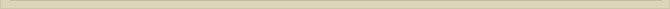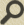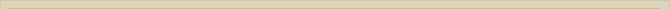## The proton and neutron

When you are interested in physics you must read “Unbelievable“!

The most well known phenomena that support the Relativity Theory have been discussed. To my knowledge every observation can rationally be explained with ether. The simplicity and transparency of the ether theory invites us to go on. It is also possible with ether to explain the existence of protons and neutrons in a similar way as the electron. We demonstrated that a positron and electron may emerge from a point-volume when the energy of the photon is high enough.

Now I ask you to imagine ether where the energy level is so high that electrons and positrons are created continuously. When a positron and electron merge both particles annihilate with two high-energy photons as result of the annihilation. Assume the density of electrons and positrons is so high that when an electron and positron merge there is no time to annihilate before other electrons and positrons present themselves to fuse. The fusing electrons and positrons add their magnetic energy to the same point-volume. The increasing magnetic spin energy forces the charge to spin in a decreasing volume. The potential energy increases accordingly. The fusion of electrons cannot go on infinitely; there must be a limit.

The energy equation for the electron at rest is: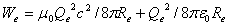When electrons and positrons fuse the energy increases proportionally. The radius of the compiled particle, according to We, will decrease proportionally. The radius will decline more and more when electrons and positrons fuse, but there will be a limit. When we fuse n+1 positrons and n electrons the formula for the total energy becomes: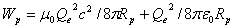with Rp=Re/(2n+1)

The magnetic and electrostatic energy is still equal. The compiled particle is 2n+1 time smaller and the energy 2n+1 time higher than the electron. It is not logical to expect that the radius can decrease to nil. There must be a limit.

The proton is, just like the electron, a very stable particle. The point-volume we described can split up into a negative and a positive point-volume, both with opposite charge Qe. About the dimensions of the point-volume there was no indication until now. Every time a positron and electron fuse, the radius declines and the energy concentration increases. The dimension of the alleged point-volume dominates the limit to the imagined fusion process.

When energy cannot concentrate anymore the fusion process will stop. This is the point where the compiled particle reaches the dimension of the presumed point-volume. The proton has emerged.

The described process is very unlikely but describes a possible physical process that gives insight how ether handles energy. It is more likely to assume that the energy concentration in the ether was so immense (Big Bang) that there was enough energy for the proton and anti-proton to emerge directly from a point-volume. With ether present in vacuum it is a coincidence that our proton is positively charged (+Qe) and not negatively. The proton could have been an anti-proton as well. In our galaxy all the nuclei are probably positive. Whether this is the case in other galaxies is to be questioned.

When we calculate the radius of the proton Rp, out of the mass: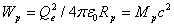With Mp the mass of the proton we calculate radius of the proton Rp at 1.535.10^-18 meter; the first indication of the dimension of the assumed point-volume.

The proton can emerge from a neutron. The neutron is not really stable. The average life span of a neutron is 900 seconds. When the neutron disintegrates, an electron and energy is ejected, and the proton  or a positron is ejected and an anti-proton emerges. Calculation of the radius Rn of a neutron in a similar way gives:

Rn=1.533.10^-18 meter

The neutron is unstable. In time it will disintegrate into a proton and electron or the counter particles: the anti-proton and positron. In the neutron the positive- and negative point volume oscillate and rotate. The neutron has not enough energy to separate in a proton and anti-proton. But there is enough energy to split it up in an electron and proton.

When we imagine the opposite process, the fusion op a proton and electron in to a neutron we are able to comprehend the properties of the neutron. Imagine a proton and electron approaching each other. The potential electrostatic energy of both particles diminishes and is transferred to kinetic energy when both particles approach.

When the electron and proton fuse electrostatic energy is transferred to kinetic/magnetic oscillation energy. After the fusion the kinetic energy has not disappeared. The kinetic energy is trapped in the neutral point-volume that emerges after both opposite charges compensate each other. The potential energy becomes kinetic energy when the particles approach. After the fusion the kinetic/magnetic energy is transferred to an electromagnetic oscillation: the oscillating positive and negative point-volume.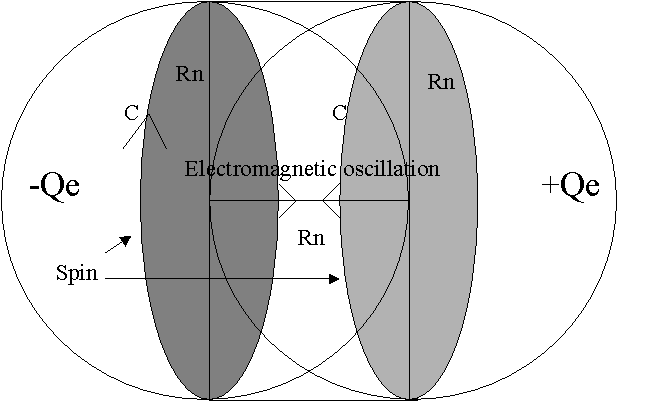Figure 20. The spinning and oscillating point-volume; the neutron.

The imagined spinning and oscillating particle, the neutron, has the energy of a proton plus 2.53 times the energy of an electron. The neutron has therefore enough energy to disintegrate in a proton and electron or an anti-proton and a positron. The neutron will be stable as long as the electromagnetic oscillation is in balance, because it has not enough energy to create a proton and anti-proton. It has however enough energy to create a proton and electron.

When the oscillation is disturbed the neutron can disintegrate. The point-volume can then separate into a positive and negative point-volume with enough kinetic and magnetic energy to become the proton (anti-proton) and electron (positron). The proton and electron are stable particles with a charge that gives them the possibility to emit overflowing energy. The neutron has no charge and can therefore not induce an electromagnetic oscillation in the ether. It is only a question of time, on average 900 seconds, before the neutron disintegrates.

When we calculate the oscillation of the positive and negative point-volume in the neutron on a classical way with the calculated radius Rn, the charges +Qe and –Qe and the energy Wn, we find that both point-volumes oscillate with the speed of light. The oscillation frequency of the neutron is so high, that the lag of the ether/vacuum surrounding the neutron prevents the ether to be polarized. The oscillation energy is preserved within the neutron.

Next chapter: The proton and neutron mathematically